# Squiggly Lines and Random Dots—You Can Fit Anything with a Nonlinear Model

(7.1)

where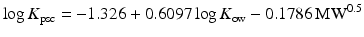(7.2)
is the permeation coefficient of the lipid fraction of the stratum corneum,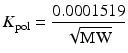(7.3)
is the permeation coefficient of the protein fraction of the stratum corneum, and(7.4)
where K ow is the octanol–water partition coefficient and MW is the molecular weight.

When Wilschut compared the original Robinson model with their iteration, they found that the original model underestimated skin permeation, and that the new model altered the influence of MW in the final model, increasing the significance of diffusion through the protein fraction of the stratum corneum.

Thus, they concluded that it was possible to make an optimum choice for a skin permeation model in connection with a specific data set (another underlying point not widely considered, but addressed albeit obliquely in “subset” studies). Their revised version of the Robinson (1993) model has the best performance (in terms of having the smallest residual variance) for the data set studied. They also commented that MW was not correctly considered in any of the models—other than Robinson’s—underlining the nonlinear nature of their analysis and of the skin permeability data set.

In more recent years, the use of nonlinear models has tended to focus on the use of Machine Learning methods, such as fuzzy logic, neural networks and Gaussian processes (GPs). The remainder of this chapter will focus on those methods, and in particular at the reasons why they appear to offer better models; why they are often criticised as being of little relevance to the real world; and why, after a only small number of publications, studies in specific areas tend to find little or no audience.

## Fuzzy Logic and Neural Network Methods for the Prediction of Skin Permeability

As described in previous chapters, models relating skin permeability to physicochemical properties of potential penetrants have classically focused on findings drawn from experimental studies. These experiments are normally in vitro models, described in Chap. 2, which involve measuring the amount of chemicals that permeate into and across skin (usually human or a suitable alternative, such as porcine skin) over a set period of time (usually 24–72 h). The amount of drug absorbed over time is determined, and from this, the flux of permeation (usually the gradient of the zero order, steady-state part of the drug release profile) is calculated. The flux and its concentration-corrected counterpart, the permeability coefficient, are commonly used to describe the process of permeation in algorithms of skin permeability. This subject is discussed in detail in earlier chapters and is described in greater depth elsewhere (Moss et al. 2002; Williams 2003; Mitragotri et al. 2013). Thus, within this chapter, the principles described in these texts are discussed not only in the context of key studies by Flynn (1990) and Potts and Guy (1992), but also in the light of Wilschut’s findings (Wilschut et al. 1995).

In general, “Machine Learning” methods were defined in 1959 by Arthur Samuel as being within a “field of study that gives computers the ability to learn without being explicitly programmed”. These methods, as applied to percutaneous absorption, are generally considered to be supervised learning methods. This is where the computer is given inputs and outputs and aims to map the former to the latter. While this encompasses most of the Machine Learning methods applied to the field of percutaneous absorption, other methods, including classification-based approaches, have also been considered; some of these methods may be categorised as unsupervised learning methods, which are more commonly used in pattern recognition studies of higher dimensional data.

One of the earliest such methods applied to the prediction of skin permeability was “fuzzy” logic. For example, Pannier et al. (2003) used the adaptive neural fuzzy interference system to model skin permeability.

Like most modelling methods, fuzzy logic essentially maps inputs to outputs. For percutaneous absorption, the output is usually the skin permeability coefficient (or perhaps the flux) and the inputs are significant physicochemical descriptors of a molecule, or a data set of molecules; commonly used descriptors include measures of lipophilicity, such as log P or log K ow, MW or molecular volume, melting point and hydrogen bond activity (i.e. the count of hydrogen bond acceptor and donor groups on a molecule). The difference in the fuzzy model is the method used to map the input to the output; independent of the methods used, all traditional modelling methods impose a mapping based on known information and a set of conventions, or rules, are used to develop the model. Such rules may include the assumed nature of the output, i.e. a linear model. An alternative to this is to use a model free from such restrictions which impose no rules on the system. In such cases, the rules are developed through the use of clustering algorithms which divide the data into natural groups, after which mapping of inputs to outputs is optimised. The rules can be either imposed by the researcher developing the method or determined from the data. It can be “crisp” (i.e. true or false statements) or “fuzzy”, where the “crispness” of the result is modified based on the nature of the data; if it lies on a continuum, it may help particular studies to avoid arbitrary cut-off [i.e. MW greater than, or less than, 150 Da, as in Flynn (1990)]. Thus, if the data have been clustered into groups where membership of each group was either partial or by degrees of belonging, as opposed to a specific “yes” or “no” to membership, then such an arrangement would be considered “fuzzy”.

Thus, Pannier et al. (2003) developed three models of skin permeability using a subtractive clustering technique, which defined structures within the data and allowed rules governing permeability to be defined. The models developed were able to predict skin permeability as well as, or better than, previously published algorithms with fewer inputs—correlation coefficients, as r2, for the three “fuzzy models” of Flynn, Potts and Guy and Abrahams, were 0.828, 0.973 and 0.959, respectively. The models developed were related to log K ow and MW (the “Flynn fuzzy model”; n = 94), and to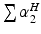and log K ow (the “Potts and Guy fuzzy model”; n = 37. The third model, the “Abraham fuzzy model”, was a variation on the Potts and Guy fuzzy model where the data set was slightly larger (n = 53) and MW was replaced by molecular volume. The authors commented that, by testing combinations of inputs, they could determine the best fuzzy model and also discern those descriptors most important to the process of skin permeability. Further, they demonstrated improvements over the traditional modelling methods and commented that further improvements in clustering methods and the range of selected inputs could improve further model quality.

Similarly, Keshwani et al. (2005) applied fuzzy logic—in this case, a rule-based Takagi-Sugeno method—to a skin permeability data set. It is interesting to note, in the context of methodological developments and the modelling of small subsets, that the authors justified the use of this method due to the “sparseness and ambiguity of available data”. They analysed a large data set (n = 140) and used lipophilicity, MW and experimental temperature (which was a combination of skin surface temperatures and water bath temperatures from a diverse range of experiments) as inputs. In comparison with simple regression methods [by comparison of r2 and root-mean-squared error (RMSE)], they found that their fuzzy model was superior, when compared with the same inputs.

It is important to note that, despite the obvious improvement in model quality and the success of such models, they have found little or no widespread use in the field of percutaneous absorption. Indeed, it is common that a small number of studies which use such methods are published which provide improved models but which may be outside the scope of dermal absorption scientists to fully apply to this field. This may be due to the lack of a defined output (an algorithm) or the technical aspects of model development [i.e. access to specific software, such as MATLAB, and to additional codes often used within such packages, as described by Pannier et al. (2003)], or to the often expensive requirement for expensive software packages.

Another successful field of sporadic interest to the modelling of percutaneous absorption is the application of artificial neural networks (ANNs). ANNs are biologically inspired computer programs which aim to mimic the perceived way in which the human brain processes information. They detect patterns and relationships within a data set and “learn”, or are trained systematically through experiential modifications, rather than from specific programming and rule development or application. ANNs are formed from numerous, often hundreds, of single processing elements (PEs) which are connected via a series of coefficients, or weightings, each of which signifies the relative importance of connections within the network (Fig. 7.1).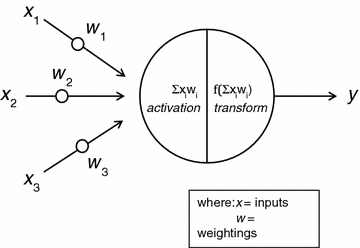Fig. 7.1
Model of an artificial neuron. From Ashrafi et al. 2015; modified from Agatonovic-Kustrin and Beresford (2000)

The inputs of each PE within a specific network are specifically weighted. They also have specific transfer, or transformation, functions and a single output (generally, in skin absorption models, this would be a prediction of the permeability coefficient). Data may feed backwards, or forwards, into different functions of the network, influencing the nature of the output (Fig. 7.2).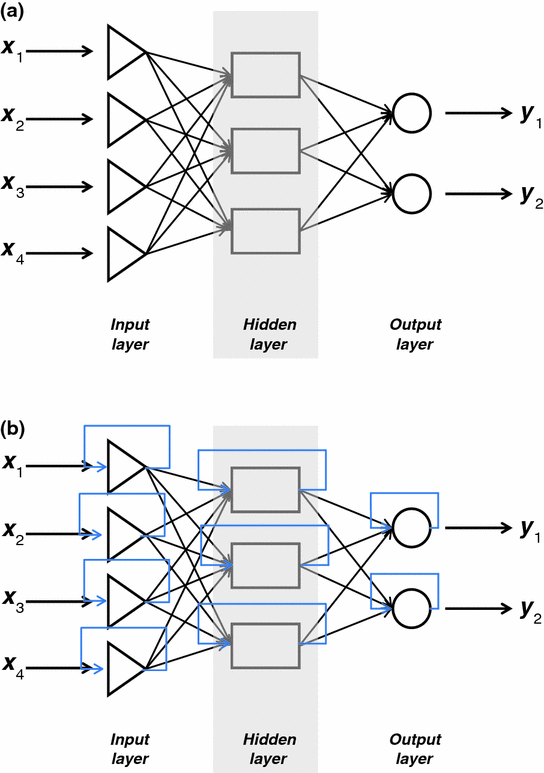Fig. 7.2
Schematic model of a feed-forward network (a, top) and a feed-backward network (b, bottom). From Ashrafi et al. 2015; modified from Agatonovic-Kustrin and Beresford (2000)

The use of transformation functions may introduce nonlinearity into the resultant model, but such phenomena are optimised for each PE within a network on order to reduce errors in predictions. Once such functions have been optimised and validated (with test and training data set, or subsets of a larger data set), then they can be used to provide predictions of skin permeability for new chemicals which are not in the original data set but which sit within its molecular space (Agatonovic-Kustric and Beresford 2000).

These methods have been widely employed in the pharmaceutical sciences; not only in modelling skin absorption but more broadly in, for example, formulation studies as an alternative to response surface methods (Agatonovic-Kustric et al. 1999), in assessing permeation across a polydimethylsiloxane membrane (Agatonovic-Kustric et al. 2001; see Chap. 5), optimisation of solid dosage form design (Bourquin et al. 1997, 1998; Takahara et al. 1998) and emulsion formulation (Alany et al. 1999; Fan et al. 2004), gene classification and protein structure prediction and sequence classification (Sun et al. 1997; Wu 1997; Milik et al. 1995). Recent research has also seen the application of genetic algorithms to pharmaceutical problem domains, specifically in the context of quantitative predictive models of drug absorption using a QSPR-based approach as described in previous chapters, where the predicted permeability across a biological membrane is related to key physicochemical descriptors of molecules in a data set (Willett 1995; So and Karplus 1996, 1997a, b). They have even been applied to clinical studies, such as the analysis of skin disease classified by Kia et al. (2013). Degim et al. (2003) applied a previously published partial charge equation and ANN methods to develop a skin permeability model. Using a data set taken from the literature (n = 40), an ANN was developed whose outputs correlated very well with experimental values (r2 = 0.997), providing a precise model for estimating percutaneous absorption (Ashrafi et al. 2015).

Chen et al. (2007) used ANNs to predict the skin permeability coefficients of novel compounds. They used a large data set (n = 215) which was described by the descriptors reported previously by Abrahams et al. (1997). Their data were subdivided into various subsets, four of which were used to train and validate the chosen models (an ANN model and a simple multiple linear regression model, which was used to benchmark the ANN model) and the remainder was used to test the models. They reported that the ANN model was nonlinear in nature and was significantly better, in terms of its statistical and predictive performance, than the linear regression model. For example, the multiple linear regression model performance was weaker in its statistical and predictive performance (n = 215; r2 = 0.699; mean-squared error (MSE) = 0.243; F = 493.556) compared to the ANN model (n = 215; r2 = 0.832; MSE = 0.136; F = 1050.653). They also concluded that the “Abrahams descriptors” were well suited to describing skin permeability, particularly in the nonlinear ANN model.

Thus, at this point, it is interesting to reflect on the nature of nonlinear models and their comparative success—in terms of statistical performance and predictive accuracy—to “Potts and Guy-type” models based on multiple linear regression methods. Such novel studies are, essentially, very similar to the classical studies in that they are based on regression or clustering/classification methods. For example, Flynn subdivided his data set into clusters based on physicochemical properties, applying distinct rules to facilitate this classification. The methods described above are essentially similar but offer more flexibility in terms of the methods of analysis, particularly nonlinear analysis, and the approach to classification and in particular boundaries, in which methods such as fuzzy logic have improved. However, in essence, the approach of such methods offers a very strong echo of Flynn’s original approach. They have also been expanded by the use of “new” descriptors, progressing from 2 parameters (lipophilicity and MW) through the adoption of the so-called Abrahams descriptors to situations where, potentially, several thousand descriptors can be determined for each member of a data set and used in its analysis. An example of this is the study by Lim et al. (2002), in which molecular orbital parameters were employed alongside more widely used descriptors to model skin absorption. They used a data set of 92 chemicals, and a number of molecular orbital terms were calculated for each member. Descriptors used included dipole moment, polarizability, the sum of charges of nitrogen and oxygen atoms and the sum of charges of hydrogen atoms bonding to nitrogen or oxygen atoms. A feed-forward back-propagation neural network model was used to analyse the data. It resulted in a model which was, statistically and in terms of predictive accuracy, better than a conventional linear model derived from multiple linear regression analysis (ANN: RMSE 0.528; linear regression: RMSE 0.930).

Nevertheless, despite a consistently superior performance to more traditional approaches—particularly multiple linear regression analysis—very few of these techniques have established themselves as first-choice methods in the prediction percutaneous absorption or even more broadly in other fields of pharmaceutical development, such as the use of ANN methods in formulation optimisation. Therefore, the real-world benefits of such methods must be assessed and their lack of uptake by pharmaceutical scientists, among others, considered.

## More Machine Learning Methods—Classification and Gaussian Process Models

In general, ANN and related Machine Learning methods require specific expertise in computer programming statistics which may be outside the reach of many physical scientists, which may impact on the ability to apply such specific and high-level applications from one field into another. In doing so, it echoes the comments by Cronin and Schultz (2003) regarding the need for specialist expertise in all aspects of model development and analysis. Indeed, this may be reflected in, for example, the work of Danick et al. (2013) in developing a spreadsheet-based model for estimating bioavailability of chemicals from dermal exposure. Implicit in such a study is the simple utility required to make a method work broadly in a different field. While some of the Machine Learning approaches suggest that Potts and Guy’s model, and the general approach of multiple linear regression, is inferior to the use of any number of Machine Learning studies, they also suggest that ease of use, transparency and broad utility that do not require specialist (and often very expensive) software are significant advantages. So too is the use of descriptors which are readily interpreted and relevant to physical scientists and which are, again, relatively straightforward to determine and which do not require expensive software packages. It would therefore appear that, currently, the limitations in Machine Learning methods outweigh their advantages. It also sends a message to those who develop and use such specific software-based approaches, which is that their utility will improve significantly if they are made more accessible and more readily interpretable by potential users in other fields.

In an example of this approach, Baert et al. (2007) employed a classification Machine Learning method to analyse a data set of 116 compounds (mostly drugs). The authors calculated and compared several models. Their initial 9-parameter multiple linear regression model only explained 40 % of the variability. They used an expanded range of computed molecular descriptors and developed a predictive algorithm based on log k p. They used a classification method—the classification and regression trees (CART) technique—which was validated by an additional twelve chemicals which were within the molecular space of their data set but not members of it. Following classification, the final model was determined by multiple linear regression analysis and resulted in a 23-term model. To avoid over-parameterisation and to simplify their model, they employed both the Kubinyi function and Akaike’s information criterion.1 Their analysis returned a q value of 9.45, well above the normal minimal value of 4 considered for the development of a linear model. Thus, they considered the inclusion of additional descriptors in their model but found that application of the Kubinyi function gave decreased values when more variables were added to the model, suggesting over-fitting. The latter test showed a biphasic asymptotic decrease, and their final model was a 10-parameter expression which the authors claimed addressed some of the concerns discussed above and presented a compromise between the statistical quality of the model, and its predictive ability as it modelled over 70 % of the variability, and its mechanistic complexity and transparency as the addition of further parameters to the model resulted only in marginal increases in its quality. Their proposed linear model is given as follows:(7.5)
where

• H.050 (atom-centred fragment) represents the number of hydrogen atoms attached to a heteroatom

• Hypertens.50 (molecular property class) is the Ghose-Viswanadhan-Wendoloski 50 % antihypertensive druglike index

• SRW09 is the self-returning walk count of order 09

• RDF075 m is the radial distribution function 7.5, which is weighted by atomic masses (i.e. the corrected probability distribution associated with finding an atom in a spherical volume with radius r)

• H.052 is the number of hydrogen atoms attached to C0(sp3) with one halogen attached to the next C

• T.(S..F) is the sum of topological distances between S and F atoms

• C.025 is the atom-centred fragment R-CR-R

• R1m+ and RTm+ are, respectively, GETAWAY class descriptors describing the maximal autocorrelation of lag 1 and the maximal index, both of which are weighted by atomic masses.

This model also had the lowest room MSE of prediction, 0.73, of the models evaluated, while the CART model had the worst (1.76). Comparison of this regression model with other published studies indicated that it was comparable in terms of its statistical quality. Thus, Baert et al. classified their data set into a distinct number of permeability classes using the CART method in order to obtain a selected number of model penetrants; this output also indicated that the OECD reference compounds caffeine, benzoic acid and testosterone were classified into different clusters. Further, models of good statistical quality were obtained using parameters that related to the lipophilic nature of penetrants and to descriptors of 3D- and 2D-molecular stereochemical complexity, and explained the skin permeability better than other descriptors. The use of the CART-clustering method indicated that, as penetrants became more lipophilic, the extra-dimensional information encoded in a three-dimensional molecular representation became less significant, while the opposite was found to be true for increasingly hydrophilic compounds.

Thus, there are several interesting outcomes from Baert’s comprehensive and excellent study. Their analysis involve the use of a wide range of descriptors, effectively employed classification/clustering techniques and expressed—and dealt with—specific concerns of over-fitting when using a wide range of descriptors; this latter point is of huge significance in the acceptance and use of nonlinear or Machine Learning methods as the general perception is that such methods will automatically over-fit data, often therefore leading to nonlinear outcomes. It also interesting to therefore note that their approach used linear regression methods to relate log k p to the significant molecular descriptors. However, the model still lacks accessibility, given the parameters returned as significant, and their utility in the field by non-experts in modelling. Thus, their approach has sadly found little further application within the field of percutaneous absorption.